# 面试必备：回溯算法详解

tony_ybliu

0收藏

1. 什么是回溯算法？
2. 一道算法题走进回溯算法
3. 回溯算法框架套路
4. leetcode案例分析
1. 什么是回溯算法
回溯算法，一种通过探索所有可能的候选解来找出所有的解的算法。

• 找到一个可能存在的正确的答案；
• 在尝试了所有可能的分步方法后宣告该问题没有答案。
举个类似的生活例子，比如放羊娃的羊在分岔路口走丟了，他顺着不同的岔路口寻找羊，一个岔路口一个岔路口的去尝试找羊。如果找不到羊，继续返回来找到岔路口的另一条路，直到找到羊为止。
如下图为找羊的决策路线图：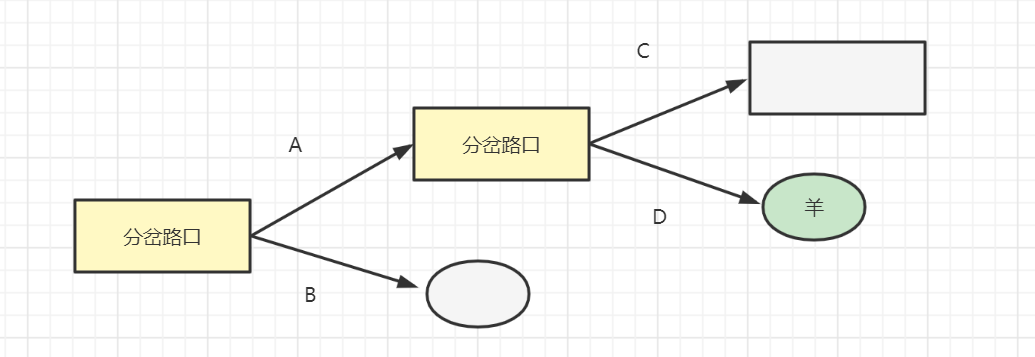2. 一道算法题走进回溯算法

``````输入：nums = [1,2,3]

``````输入：nums = [0,1]

2.1 实现思路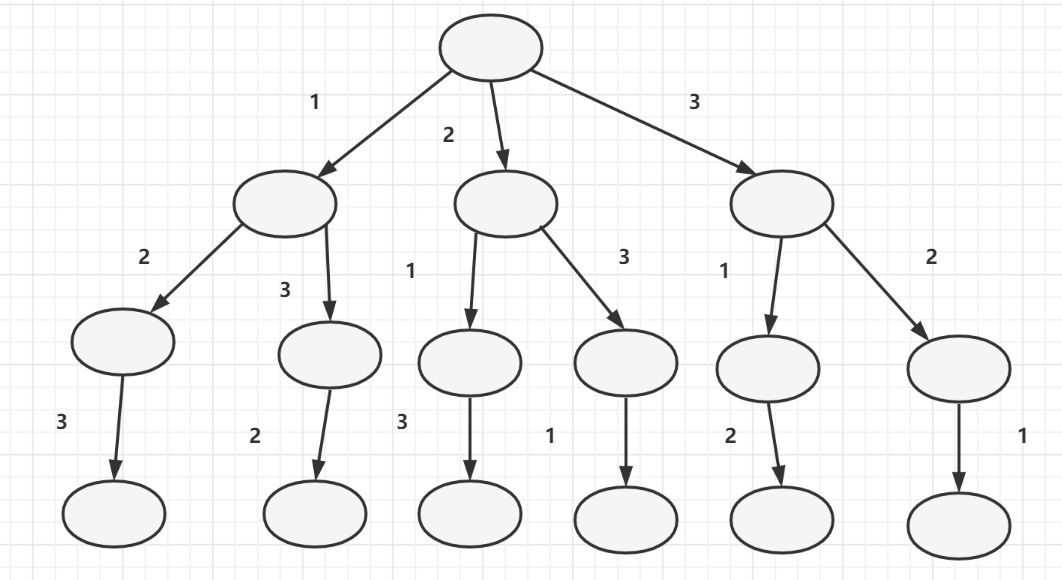• 为了方便理解，我们可以把nums个数k看做k种选择，比如对于[1,2,3]，每一位都有3种选择：1、2、3。
• 每一次做选择，都展开出一棵空间树，
• 选择完后，如果是重复选的路径，就做剪枝。
上图的那颗树，可以看做是遍历所有元素，展开空间树，然后剪枝得来的。如下图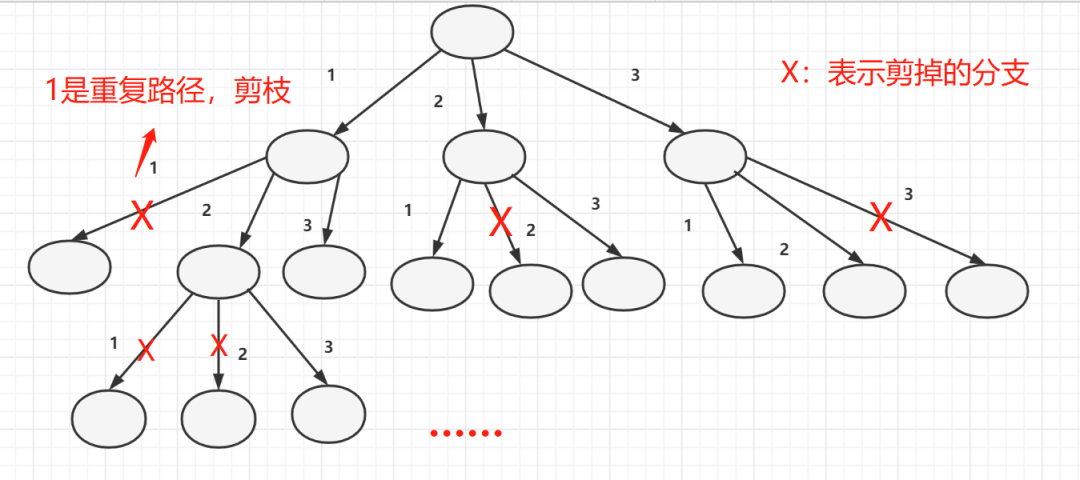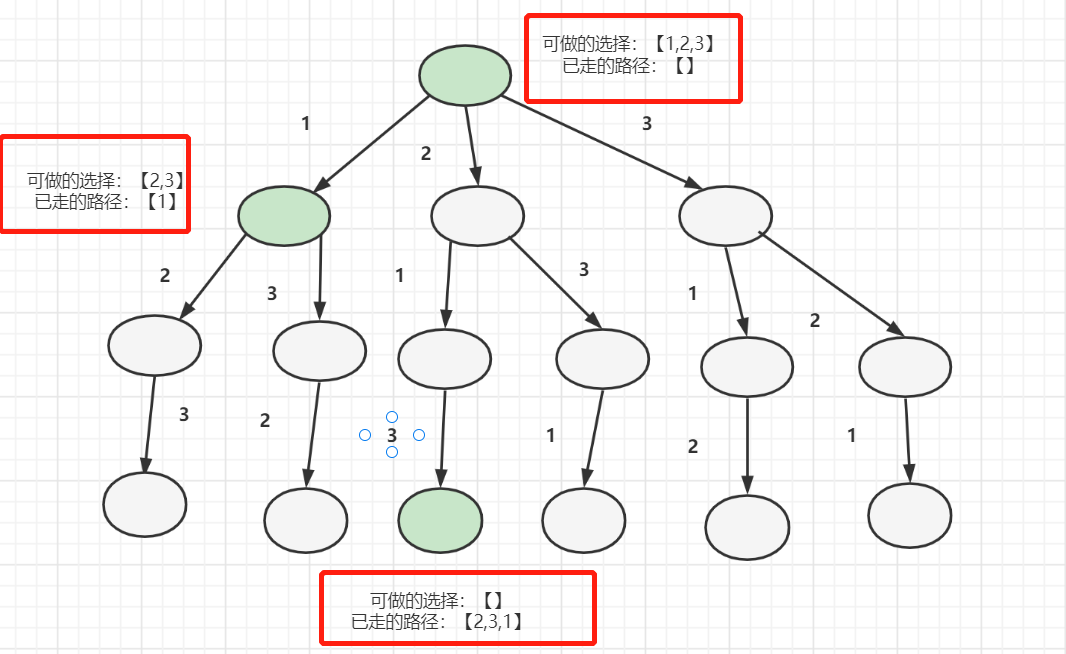2.2 代码实现

• 递归入口是什么呢？一个可选路径和已走过的路径就好啦。
• 递归函数体呢？一个for循环，枚举当前数组的元素，并且需要if判断，以跳过剪枝
• 递归出口呢？也就是走到叶子节点啦，叶子节点，就是当构建的已走路径path的数组长度等于nums的长度
实现代码如下：
``````class Solution {
//全排列，即所有路径集合

public List<List<Integer>> permute(int[] nums) {
//当前路径，入口路径，path是空的
//递归函数入口，可做选择是nums数组
backTrace(nums,path);
return allPath;
}

public void backTrace(int[] nums,List<Integer> path){
//已走路径path的数组长度等于nums的长度,表示走到叶子节点，所以加到全排列集合
if(nums.length==path.size()){
return;
}

for(int i=0;i<nums.length;i++){
//剪枝，排查已经走过的路径
if(path.contains(nums[i])){
continue;
}
//做选择，加到当前路径
//递归，进入下一层的决策
backTrace(nums,path);
//取消选择
path.remove(path.size() - 1);
}
}
}``````

• 因为我们不是要找到一个排列就好了，而是需要找出所有满足条件的排列
• 当递归调用结束时，结束的是当前的递归分支，还需要去别的分支继续找
• 因此需要撤销当前的选择，回到选择前的状态，再选下一个选项，即进入下一个分支。
3. 回溯算法框架套路
穷举找规律，总结出回溯决策树
套用回溯算法框架代码
3.1. 穷举找规律，总结出回溯决策树
回溯类型问题的问题，基础也是穷举。我们一般通过穷举找到规律，然后画出回溯决策树就好啦。比如以上全排列的例子。

3.2. 套用回溯算法框架

• 已走路径：已做出选择，走过的路径
• 可选列表：你当前可以做的选择
• 结束条件：一般走到决策树的叶子节点，它无法再做别的条件选择
回溯算法伪代码框架如下：
``````//所有路径集合
List<> allPath  = []
void backTrace (可选列表，已走路径)：
if(满足结束条件){
return;
}
for(选择：可选列表){
做选择
backTrace（当前可选列表，已走路径）;
撤销选择
}``````

4. leetcode案例分析

candidates中的同一个数字可以无限制重复被选取。如果至少一个数字的被选数量不同，则两种组合是不同的。

``````输入：candidates = [2,3,6,7], target = 7

2 和 3 可以形成一组候选，2 + 2 + 3 = 7 。注意 2 可以使用多次。
7 也是一个候选， 7 = 7 。

``````输入: candidates = [2,3,5], target = 8

4.1 思路

``````7 = 2 + 2 + 3
7 = 7``````

``````8 = 2+ 2 + 2 +2
8 = 2 + 3 + 3
8 = 3 + 5``````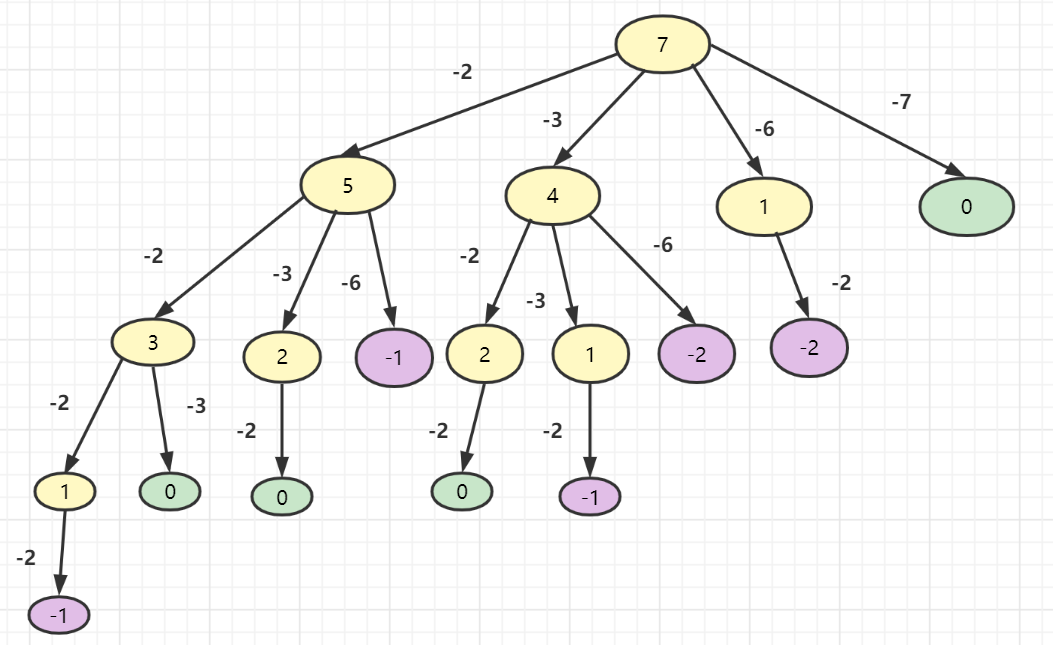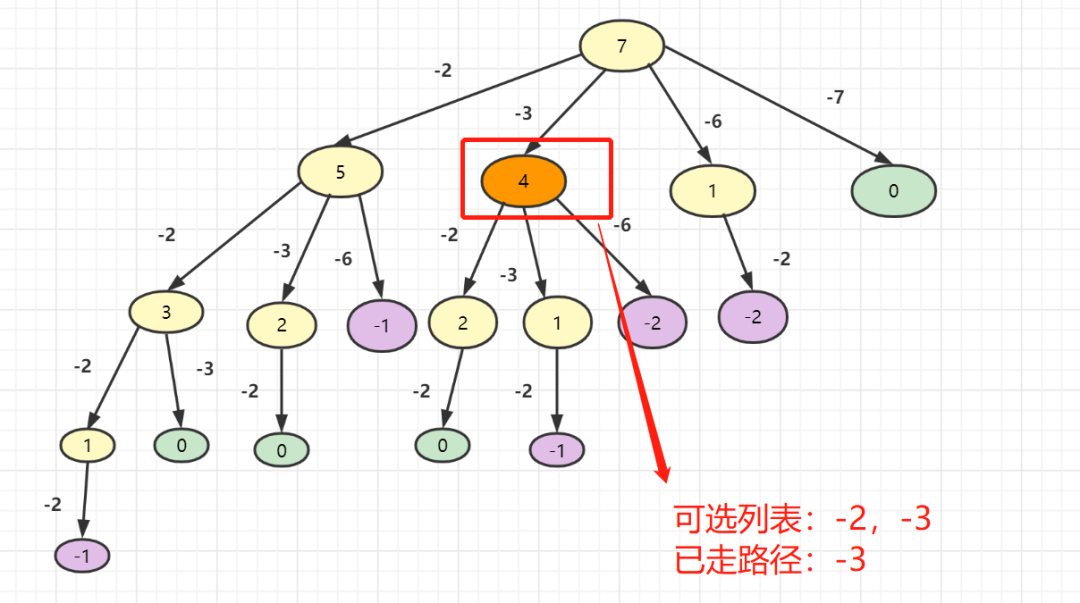4.2 代码实现

``````class Solution {

public List<List<Integer>> combinationSum(int[] candidates, int target) {
backTrace(candidates,target,path,0);
return allPath;
}

public void backTrace(int[] candidates,int target, List<Integer> path,int start){

if (target < 0) {
return ;
}
//满足结束条件，加到总路径
if(target==0){
return;
}

for (int i = start; i < candidates.length; i++) {
//可选列表：当前结点大于要走的路径数值
if (target >= candidates[i]) {
//做选择
//递归
backTrace(candidates, target - candidates[i], path, i);
//撤销选择
path.remove(path.size() - 1);
}

}
}
}``````

• leetcode官网